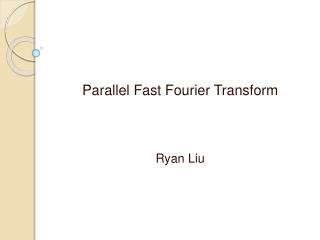DownloadDownload PresentationParallel Fast Fourier Transform Ryan Liu

# Parallel Fast Fourier Transform Ryan Liu

Download Presentation## Parallel Fast Fourier Transform Ryan Liu

- - - - - - - - - - - - - - - - - - - - - - - - - - - E N D - - - - - - - - - - - - - - - - - - - - - - - - - - -
##### Presentation Transcript

1. Parallel Fast Fourier Transform Ryan Liu

2. Introduction • The Discrete Fourier Transform could be applied in science and engineering. • Examples: • Voice recognition • Image processing • Discrete Fourier Transform (DFT): O(n2) • Fast Fourier Transform (FFT): O(n log n)

3. Fourier Analysis • Fourier analysis: Represent continuous functions by potentially infinite series of sine and cosine functions. • Fourier Series: A function can be expressed as the sum of a series of sines and cosines:

4. Fourier Transform • Fourier Series can be generalized to derive the Fourier Transform • Forward Fourier Transform: • Inverse Fourier Transform: Note:

5. Fourier Transform • Fourier Transform maps a time series into the series of frequencies that composed the time series. • Inverse Fourier Transform maps the series of frequencies back into the corresponding time series. • The two functions are inverses of each other.

6. Discrete Fourier Transform • Maps a sequence over time to another sequence over frequency. • The Discrete Fourier Transform (DFT): k = 0, … N -1 n = 0, … N -1 Let k represent the discrete time signal, and Fn represent discrete frequency transform function.

7. Speech example of DFT “Angora cats are furrier…” Signal Frequency and amplitude

8. DFT Computation • n elements vector x. • DFT matrix vector product Fnx • fi,j = wnij for , j < n, and wn is the primitive nth root of unity.

9. DFT Example1 • DFT of vector (2,3) • The primitive square root of unity for w2 is -1 • The inverse of DFT

10. DFT Example2 • DFT of vector (1,2,4,3) • The primitive 4th root of unity for w4 is i

11. DFT Example2 • Inverse DFT

12. Fast Fourier Transform • DFT requires O(n2) time to process for n samples: • So, using DFT is not a best way in practice. • Fast Fourier Transform: • Produces exactly the same result as the DFT. • Time complexity O(n log(n)). • divide-and-conquer strategy.

13. FFT • Recursively breaks down a DFT of any composite size N = N1N2 into many smaller DFTs of sizes N1 and N2, along with O(N) multiplication. • It’s to divide the transform into two pieces of size N/2 at each step.

14. Parallel FFT • Algorithm: recursive FFT(1,2,4,3) FFT(1,4) FFT(2,3) FFT(1) FFT(4) FFT(2) FFT(3)

15. Parallel FFT • Tracking the flow of data values.

16. Phases of Parallel FFT • Phase 1: Processes permute the input sequence. • Phase 2: • First log n – log p iterations of FFT • No message passing is required • Phase 3: • Final log p iterations • In each iteration every process swaps values with partner.

17. Time Complexity Analysis • FFT time complexity O(n log n). • Parallel FFT • Each process controls n/p elements • The overall communication time complexity is O( (n/p) log p) • Computational time complexity of parallel FFT is O(n log n/p)

18. Reference • Quinn,M.J (2004). Parallel programming in C with MPI and OpenMP • Chu, E., & George. A., (2000). Serial and Parallel Fast Fourier Transform Algorithms • Bi,G & Zeng, Y. (2003). Transforms and Fast Algorithm for Signal Analysis and Representation. • The Fast Fourier Transform. (n.d.). Retrieved from http://www.dspguide.com/ch12/2.htm • Chu, E., & George, A., (1999). Inside the FFT black box: serial and parallel fast Fourier transform algorithms. Boca Raton, Fla.: CRC Press, 1999.